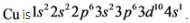# Express the complete electronconfiguration using superscripts where appropriate

Part A
Give the ground-state electron configurationfor silicon (Si).
Express the complete electronconfiguration using superscripts where appropriate. For example,the configuration for Li would be entered as1s^22s^1.

Part B
Give the actual ground-stateelectron configuration for copper (Cu).
Express the electron configurationusing superscripts where appropriate. For example, theconfiguration for Li would be entered as 1s^22s^1.

Concepts and reason
The problem is based on the concept of electronic configuration of elements.
The distribution of electrons in an orbital is represented by the electronic configuration. The electrons are filled in increasing order of energy.

Fundamentals
Electronic configuration represents the distribution of electrons in the orbital shells and subshells. The electrons are arranged in the increasing order of principal quantum number. While filling the electrons in an orbital they follow Pauli’s Exclusion principle, Hund’s Rule and Aufbau’s Principle. As per Pauli’s Exclusion principle, no two electrons can have the same quantum number. Hund’s rule states that electron will singly occupy the orbital in the subshell with the same spin. Aufbau’s principle state that electrons are filled in the increasing order of energy.

Part A
Silicon atom, Si has 14 electrons. Therefore, the electronic configuration of Silicon is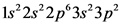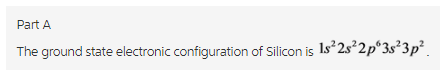Explanation:
Silicon atom has 14 electrons. The electrons are filled in the increasing order of energy. Therefore, the increasing order is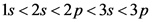. The s-orbital can have maximum 2 electrons while the p orbital can hold up to 6 electrons. Therefore, the ground state electronic configuration of Silicon is.

Part B
Copper atom has 29 electrons. Therefore, the electronic configuration of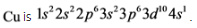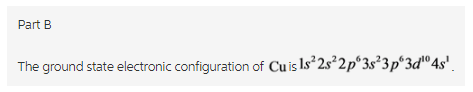Explanation:
Copper atom has 29 electrons. The electrons are filled in the increasing order of energy. Therefore, the increasing order is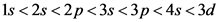. s-orbital can have maximum 2 electrons, p orbital can hold up to 6 electrons while d-orbital can hold up to 10 electrons. When the orbital is half –filled or fully filled, it gives stability to the atom. Therefore, the ground state electronic configuration of